Julian Gilbey

•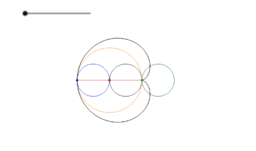Rolling on a cycloid

Activity

Julian Gilbey

•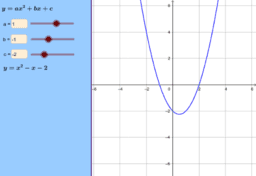Activity

Julian Gilbey

•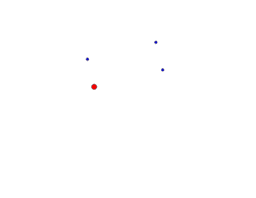Four dots

Activity

Julian Gilbey

•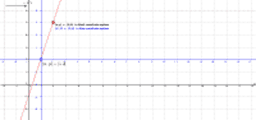Equations of straight line graphs via changing coordinates

Activity

Julian Gilbey

•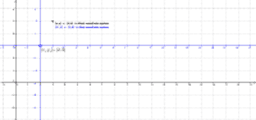The effect of changing coordinate systems on a point

Activity

Julian Gilbey

•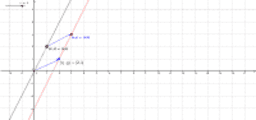Translating straight lines to find their equations

Activity

Julian Gilbey

•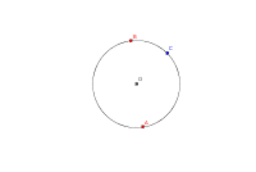Centre of a circle passing through two fixed points

Activity

Julian Gilbey

•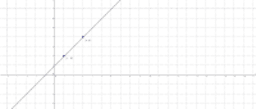Straight line with given gradient through a specific point

Activity

Julian Gilbey

•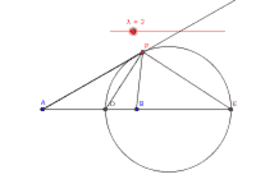The Circle of Apollonius

Activity

Julian Gilbey

•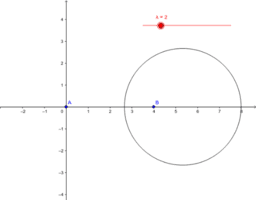The Circle of Apollonius

Activity

Julian Gilbey

•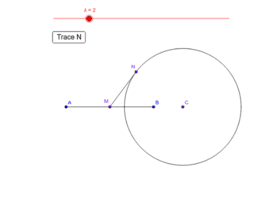A tangent to the circle of Apollonius

Activity

Julian Gilbey

•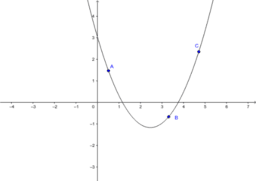Activity

Julian Gilbey

•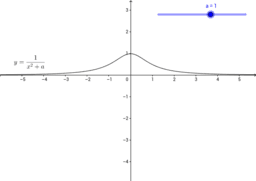Activity

Julian Gilbey

•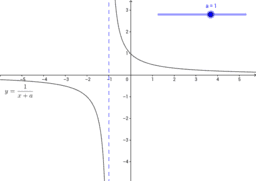Reciprocal linear function graphs

Activity

Julian Gilbey

•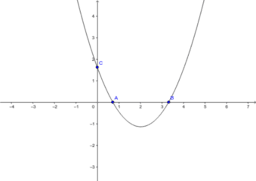Constructing a quadratic through three constrained points

Activity

Julian Gilbey

•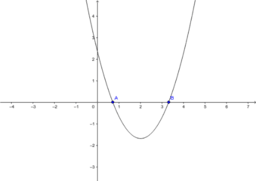Constructing a quadratic through two points

Activity

Julian Gilbey

•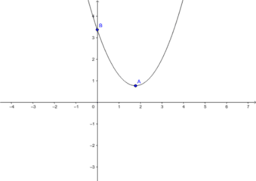A quadratic passing through specified points

Activity

Julian Gilbey

•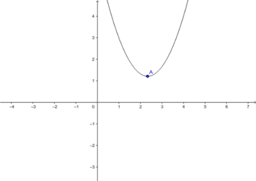A quadratic with its vertex at a specified point

Activity

Julian Gilbey

•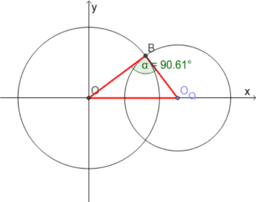Intersecting circles

Activity

Julian Gilbey

•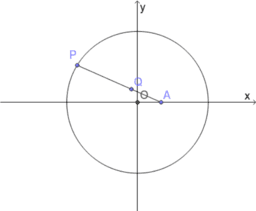Locus of a point on a line from a fixed point to a circle

Activity

Julian Gilbey

•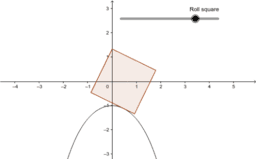Roulette: Rolling a square on a catenary

Activity

Julian Gilbey

•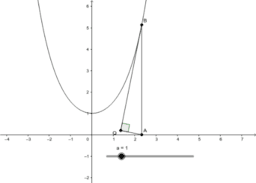The Tractrix and the Catenary

Activity

Julian Gilbey

•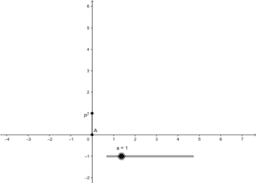The Tractrix

Activity

Julian Gilbey

•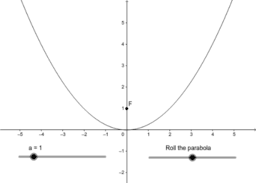Rolling a parabola

Activity

Julian Gilbey